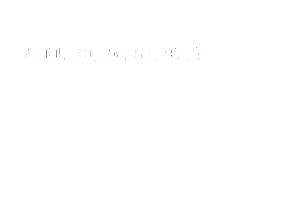Home > Math Shortcuts > Brain Trigger #43 – Sample Number puzzle question

# Brain Trigger #43 – Sample Number puzzle question

Now here we will discuss few question answers on Number puzzle which are very common in competitive exams. These types of Number puzzle questions will help you to prepare for your exam. Try to Solve these questions with your math skills. These Number puzzle questions can also be solved using math shortcut tricks.

We try our best to get all type of shortcut tricks to solve these problems and bring them together here in this website. All you have to do is now to apply those shortcut tricks here to solve these Number puzzle problems. You can solve these questions without using any shortcut tricks also.

Here we will discuss a question on Number puzzle. All you need to do is to read the question very carefully and try to solve it by yourself. We will provide the answer of this question below. If you do it yourself then check your answer with our solution. You can also check below if you don’t know how to do the problem correctly.

Each page contains a question on Number puzzle and also its solution with explanation. Click on the Next/Previous link to navigate through other questions.

Let’s begin question with answer session. Today’s question is:

What should be the number replaces in the given series ?Scroll down below to know the answer of this question. Take as much time as you want to get the answer of this question. But try to solve this as quicker as you can.

(Scroll down to get the answer)

(Scroll down to get the answer)

(Scroll down to get the answer)

(Scroll down to get the answer)

Here starts the solution of the previous problem. If you already done this by yourself then please check your answer with us. A very Well Done if your answer is correct, but don’t worry if you are not able to answer the question correctly. Here is the solution of the problem with explanation.

You can solve in this way……….

+22, +32, +42, +52, +62, +72,
add each square number to getting next number of this series….
So, the out come relust of this series is 146.